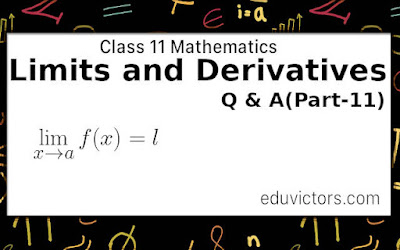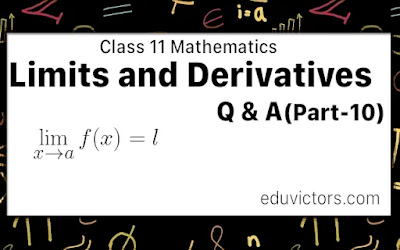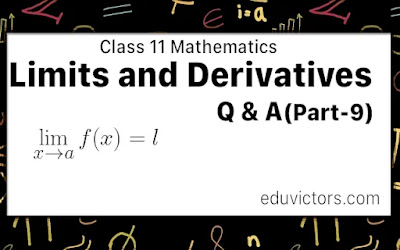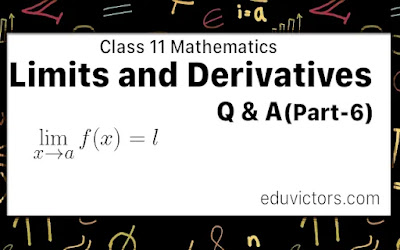## Pages

Showing posts with label calculus. Show all posts
Showing posts with label calculus. Show all posts

# Class 12 - Mathematics - Differential Equations - Part - 1

An equation involving an independent variable (variables), dependent variable and derivative or derivatives of the dependent variable with respect to the independent variable (variables) is called a differential equation.

It is an equation involving unknown functions and their derivatives w.r.t. one or more independent variables.# Methods of Differentiation

CBSE Class 11 - Mathematics - Limits and Derivatives Part-11

Working rule for finding the derivative of implicit functions

First method:

Differentiate every term of f (x, y) = 0 with respect to x.# DIFFERENTIATION OF STANDARD FUNCTIONS

CBSE Class 11 - Mathematics - Limits and Derivatives Part-10

You have learnt theorems of differentiation part-9. Let us proceed further. Here is a list of differentiation of some standard functions:# THEOREMS ON DIFFERENTIATION

CBSE Class 11 - Mathematics - Limits and Derivatives Part-9

You have learnt about finding derivatives using the first principle in part-7 and part-8. Let us proceed further. Here is a summary of theorems on differentiation.# CBSE Class 11 - Mathematics - Limits and Derivatives Part-7

Derivatives Using First Principle

In the previous post[Part-6], we learned about the Derivative of a function at a point. Let us focus on some important derivatives using the First Principle.

Theorem 1: From the first principle, we have# Class 11 - Mathematics - Limits and Derivatives (Part-6) - Derivative of a Function At a Point

In the previous blog post Limits and derivatives Part-5, we learned about the limits. Let us understand the derivative of a function at a point.

Q1: What is the derivative of a function at a point?

Answer: Let y = f(x) is a continuous function. It means the value of y changes as the value of x changes.

At x = a, is a point in its domain of definittion. The derivative of f at a is defines as: